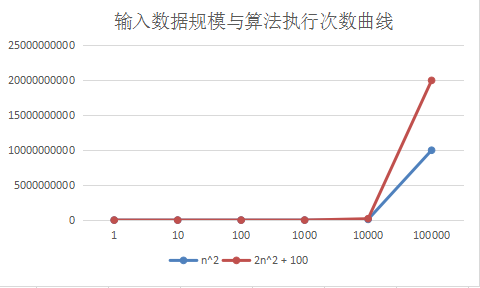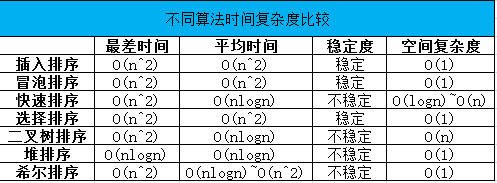2019/04/10 10:10

# 1. 时间复杂度

## 1.4 举例说明

1. T(n) = 2: 该函数总共执行两条语句，所以基本执行次数为2；时间复杂度为O(1): 该函数的基本执行次数只有常数项，所以时间复杂度为O(1)
void test(int n)
{
int a;
a = 10;
}

1. T(n) = 2n: 该函数共循环n次，每次执行2条语句，所以基本执行次数为2n。时间复杂度舍弃系数，为O(n)
void test(int n)
{
int cnt;
for (cnt = 0; cnt < n; cnt++) {
int a;
a= 10;
}
}

1. T(n) = 2 * (1 + 2 + 3 + 4 + ... + n) + 1 = 2 * (1 + n) * n / 2 + 1 = n^2 + n + 1。因为共执行(1 + 2 + 3 + 4 + ... + n) 次循环，每次循环执行2条语句，所有循环结束后，最后又执行了1条语句，所以执行次数如上；时间复杂度为O(n^2)，因为n和常数项1忽略，它们在数据量剧增的时候，对于执行次数曲线几乎没有影响了
void test(int n)
{
int cnt1, cnt2;
for (cnt1 = 0; cnt1 < n; cnt1++) {
for (cnt2 = cnt1; cnt2 < n; cnt2++) {
int a;
a = 10;
}
}
a = 11;
}

1. T(n) = 2 * logn 因为每次循环执行2条语句，共执行logn次循环；时间复杂度为O(logn)，忽略掉系数2
void test(int n)
{
int cnt;
for (cnt = 1; cnt < n; cnt *= 2) {
int a;
a = 10;
}
}

1. T(n) = n * logn * 2 因为每次循环2条语句，共执行n * logn次循环；时间复杂度为O(nlogn)，忽略掉系数2
void test(int n)
{
int cnt1, cnt2;
for (cnt1 = 0; cnt1 < n; cnt1++) {
for (cnt2 = 1; cnt2 < n; cnt2 *= 2) {
int a;
a = 10;
}
}
}

1. T(n) = 2 * n^3 因为每次循环2条语句，共执行n^3 次循环；时间复杂度为O(n^3)，忽略掉系数2
void test(int n)
{
int cnt1, cnt2, cnt3;
for (cnt1 = 0; cnt1 < n; cnt1++) {
for (cnt2 = 0; cnt2 < n; cnt2++) {
for (cnt3 = 0; cnt3 < n; cnt3++) {
int a;
a = 10;
}
}
}
}

1. 斐波那契数列的递归实现，每次调用该函数都会分解，然后要再调用2次该函数。所以时间复杂度为O(2^n)
int test(int n)
{
if (n == 0 || n == 1) {
return 1;
}
return (test(n-1) + test(n-2));
}


## 1.5 时间复杂度比较

O(1) < O(log2n) < O(n) < O(nlog2n) < O(n^2) < O(n^3) < O(2^n) < O(n!) < O(n^n)

# 2. 空间复杂度

## 2.1 概念

• 程序本身所占用的空间
• 输入输出变量所占用的空间
• 动态分配的临时空间，通常指辅助变量

## 2.2 举例说明

1. S(n) = O(1).空间复杂度为O(1)，因为只有a, b, c, cnt四个临时变量。且临时变量个数和输入数据规模无关。
int test(int n)
{
int a, b, c;
int cnt;
for (cnt = 0; cnt < n; cnt++) {
a += cnt;
b += a;
c += b;
}
}

1. S(n) = O(n).空间复杂度为O(n)，因为每次递归都会创建一个新的临时变量a。且共递归n次。
int test(int n)
{
int a = 1;
if (n == 0) {
return 1;
}
n -= a;
return test(n);
}


# 3. 函数的渐进增长与渐进时间复杂度# 4. 不同算法的时间复杂度 & 空间复杂度## 感谢大家的阅读，大家喜欢的请帮忙点下推荐。后面会继续出精彩的内容，敬请期待！

==转载请在显著位置注明：==

<center>CrazyCatJack</center>

0
0 收藏

### 作者的其它热门文章0 评论
0 收藏
0